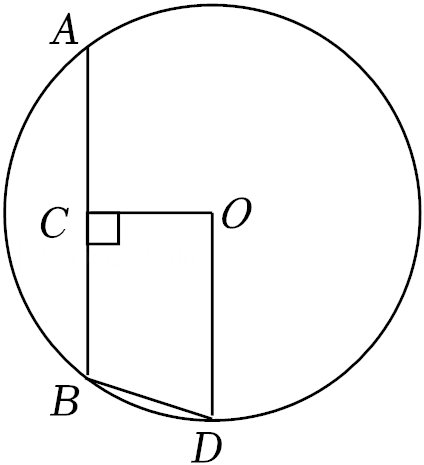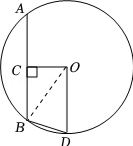$\text{A.}$ $90^{\circ}$ $\text{B.}$ $95^{\circ}$ $\text{C.}$ $100^{\circ}$ $\text{D.}$ $105^{\circ}$
【答案】 D

【解析】\begin{aligned} & \because \mathrm{OC}=\frac{1}{2} \mathrm{OD}, \\ & \therefore \mathrm{OC}=\frac{1}{2} \mathrm{OB}, \\ & \because \mathrm{OC} \perp \mathrm{AB}, \\ & \therefore \angle \mathrm{OBC}=30^{\circ}, \\ & \because \mathrm{OD} / / \mathrm{AB}, \\ & \therefore \angle \mathrm{BOD}=\angle \mathrm{OBC}=30^{\circ}, \\ & \therefore \angle \mathrm{OBD}=\angle \mathrm{ODB}=75^{\circ}, \\ & \angle \mathrm{ABD}=30^{\circ}+75^{\circ}=105^{\circ} . \end{aligned}